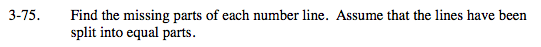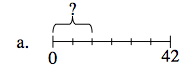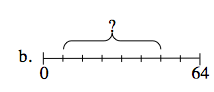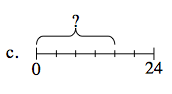### Home > MC2 > Chapter 3 > Lesson 3.2.2 > Problem3-75

3-75.How many segments is 42 broken into? Use this to figure out the value of each segment.How many segments is 64 broken into? Use this to figure out the value of each segment.

40. Be sure to show why this answer is correct.How is this problem the same or different than parts (a) and (b)?